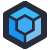Krzysztof found themselves staring at a C++ function called `intToString`. And staring. And then realized that the function makes more sense if you don't look at the name. Let's take a peek:

``````String intToString(u8* p_param, int p_length) {
int i;
int j=0;
for(i=0; i < p_length; i++)
{
j = p_param[i];

if(j==97 || j==98 || j==99 || j==100 || j==101 || j==102 || j==103 || j==104 ||
j==105 || j==106 || j==107 || j==108 || j==109 || j==110 || j==111 || j==112 ||
j==113 || j==114 || j==115 || j==116 || j==117 || j==118 || j==119 || j==120 ||
j==121 || j==122)
{
p_param[i] = j-32;
}

if(!isprint((char)p_param[i]))
p_param[i] = 0;
}

string s((char*) p_param);
return s;
}
``````

So, `intToString` takes a buffer of bytes and a length for the buffer, and at a glance, you'd just assume that someone is reinventing `atoi`. We go into the loop, and check each character, and someone has opted to unroll a basic range comparison into a long series of or operations. Which is dumb, but… wait a second. ASCII character 97… we subtract 32…

This function isn't an `intToString` function. It's a "`toUpper` function. Also an existing function that didn't need to be reinvented, also a case where we didn't need to unroll the `if` comparison so hard.

It's also worth noting that `String` is a typedef for `std::string`, which isn't wrong but leaves us asking "why?"[Advertisement] ProGet’s got you covered with security and access controls on your NuGet feeds. Learn more.